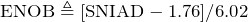# Increasing SQNR by Increasing Bits Per Sample

December 3, 2019

Increasing the number of bits per sample will decrease the quantization noise and, therefore, increase SQNR. Theorem 3 quantifies this qualitative relationship for a full-scale sine waveform input. Take note that the SQNR increases approximately 6dB for every additional bit.

### Theorem 3

Let SQNR be the signal to quantization noise ratio for a full-scale sinusoid input.

Let b be the number of bits used by a converter. Then:

(1)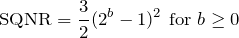(2)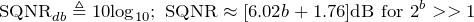#### Proof

(1) The power Ps of a sine waveform Asin(2𝜋𝑓 𝑡 + 𝜙) is A2/2

Proof: Let 𝜏 = 1/𝑓c  be one period of the sine waveform.

(3)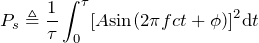(4)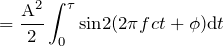(5)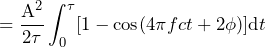by half-angle formula/squared identity (6)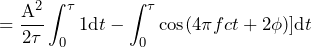by half-angle formula/squared identity

(7)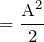(2) For SQNR:

 (8)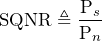by definition of SQNR (9)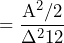by item (1) above and the proposition Var v(n) (10)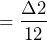from the lesson Quantization and Noise (11)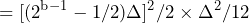from the lesson Quantization and Noise

(12)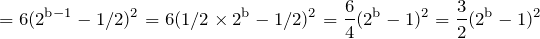(3) For SQNRdB:

 (13)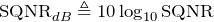by the definition of SQNRdB (14)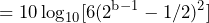by proof (2) (15)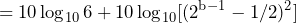by property of logarithms (16)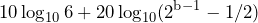by property of logarithms (17)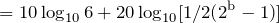(18)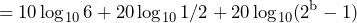by property of logarithms (19)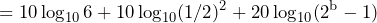by property of logarithms (20)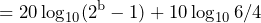by property of logarithms (21)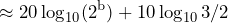for 2b>>1 (22)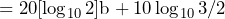by property of logarithms (23)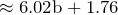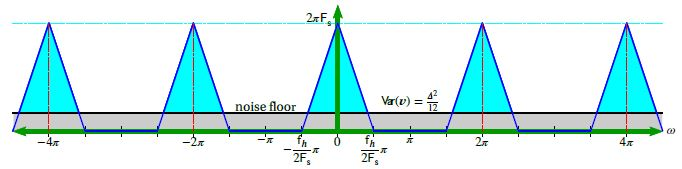### Over-Sampling with Quantization Uniformly Distributed White Noise

Theorem 3 is a theoretical ideal. In practice, an N-bit converter has issues with inherent noise and doesn’t provide N bits of precision. Given this, system engineers want to know how many effective bits they can achieve. The b in Theorem 3 is equated in terms of SQNRdB to yield the following quantity called ENOB.

Let SINAD be the signal-to-noise-and-distortion ratio. Then:

(24)